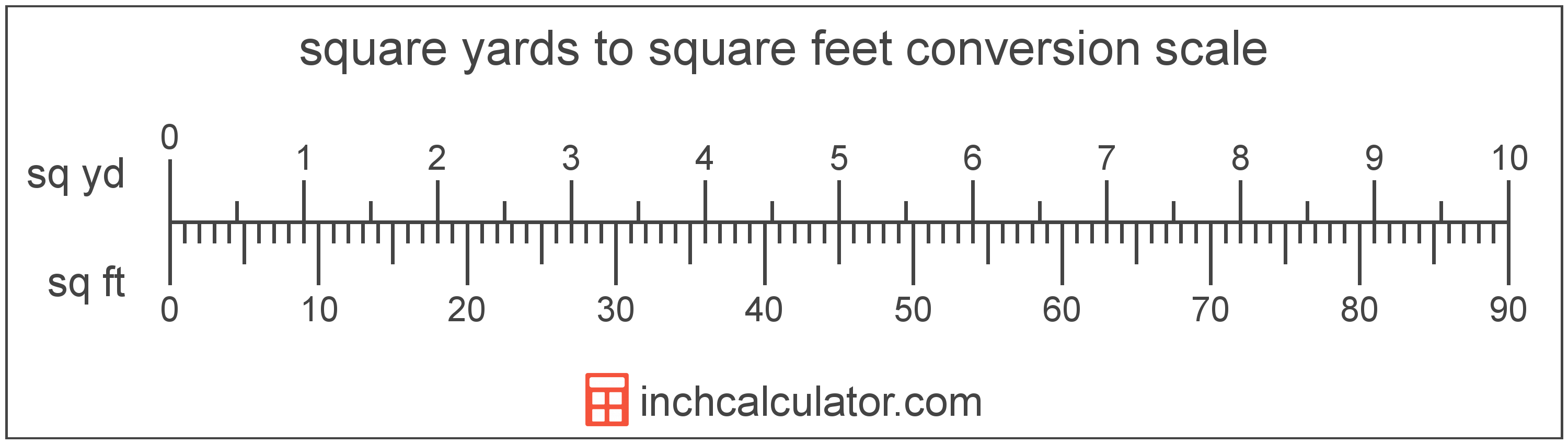# Square Feet to Square Yards Converter

Enter the area in square feet below to get the value converted to square yards.

(find square feet)
Results in Square Yards:9 sq ft = 1 sq yd

Do you want to convert square yards to square feet?

## How to Convert Square Feet to Square Yards

To convert a measurement in square feet to a measurement in square yards, divide the area by the following conversion ratio: 9 square feet/square yard.

Since one square yard is equal to 9 square feet, you can use this simple formula to convert:

square yards = square feet ÷ 9

The area in square yards is equal to the area in square feet divided by 9.

For example, here's how to convert 5 square feet to square yards using the formula above.
square yards = (5 sq ft ÷ 9) = 0.555556 sq yd### How Many Square Yards Are in a Square Foot?

There are 0.111111 square yards in a square foot, which is why we use this value in the formula above.

1 sq ft = 0.111111 sq yd

## What Is a Square Foot?

One square foot is equivalent to the area of a square with sides that are each 1 foot in length. One square foot is equal to 144 square inches or 0.092903 square meters .

The square foot is a US customary and imperial unit of area. A square foot is sometimes also referred to as a square ft. Square feet can be abbreviated as sq ft, and are also sometimes abbreviated as ft². For example, 1 square foot can be written as 1 sq ft or 1 ft².

You can use a square footage calculator to calculate the area of a space if you know its dimensions.

## What Is a Square Yard?

One square yard is equivalent to the area of a square with sides that are each 1 yard in length. One square yard is roughly equal to 9 square feet or 0.836127 square meters.

The square yard is a US customary and imperial unit of area. A square yard is sometimes also referred to as a square yd. Square yards can be abbreviated as sq yd, and are also sometimes abbreviated as yd². For example, 1 square yard can be written as 1 sq yd or 1 yd².

You can use a square yards calculator to calculate the area of a space if you know its dimensions.

## Square Foot to Square Yard Conversion Table

Table showing various square foot measurements converted to square yards.
Square Feet Square Yards
1 sq ft 0.111111 sq yd
2 sq ft 0.222222 sq yd
3 sq ft 0.333333 sq yd
4 sq ft 0.444444 sq yd
5 sq ft 0.555556 sq yd
6 sq ft 0.666667 sq yd
7 sq ft 0.777778 sq yd
8 sq ft 0.888889 sq yd
9 sq ft 1 sq yd
10 sq ft 1.1111 sq yd
11 sq ft 1.2222 sq yd
12 sq ft 1.3333 sq yd
13 sq ft 1.4444 sq yd
14 sq ft 1.5556 sq yd
15 sq ft 1.6667 sq yd
16 sq ft 1.7778 sq yd
17 sq ft 1.8889 sq yd
18 sq ft 2 sq yd
19 sq ft 2.1111 sq yd
20 sq ft 2.2222 sq yd
21 sq ft 2.3333 sq yd
22 sq ft 2.4444 sq yd
23 sq ft 2.5556 sq yd
24 sq ft 2.6667 sq yd
25 sq ft 2.7778 sq yd
26 sq ft 2.8889 sq yd
27 sq ft 3 sq yd
28 sq ft 3.1111 sq yd
29 sq ft 3.2222 sq yd
30 sq ft 3.3333 sq yd
31 sq ft 3.4444 sq yd
32 sq ft 3.5556 sq yd
33 sq ft 3.6667 sq yd
34 sq ft 3.7778 sq yd
35 sq ft 3.8889 sq yd
36 sq ft 4 sq yd
37 sq ft 4.1111 sq yd
38 sq ft 4.2222 sq yd
39 sq ft 4.3333 sq yd
40 sq ft 4.4444 sq yd

## References

1. Merriam-Webster, square foot, https://www.merriam-webster.com/dictionary/square%20foot